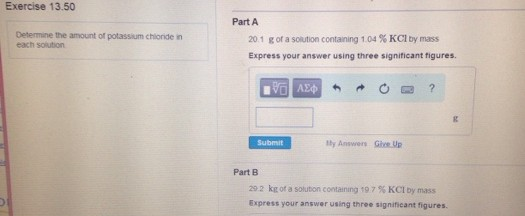# Problem: Determine the amount of potassium chloride in each solution. 20.1 g of a solution containing 1.04 % KCl by mass. Express your answer using three significant figures. 29.2 kg of a solution containing 19.7 % KCl by mass. Express your answer using three significant figures.

###### FREE Expert Solution
79% (267 ratings)###### Problem Details

Determine the amount of potassium chloride in each solution.

20.1 g of a solution containing 1.04 % KCl by mass. Express your answer using three significant figures.

29.2 kg of a solution containing 19.7 % KCl by mass. Express your answer using three significant figures.